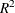# The SYSLIN Procedure

### OLS Estimation

PROC SYSLIN performs OLS regression if you do not specify a method of estimation in the PROC SYSLIN statement. OLS does not use instruments, so the ENDOGENOUS and INSTRUMENTS statements can be omitted.

The following statements estimate the supply and demand model shown previously:

```proc syslin data=in;
demand: model q = p y s;
supply: model q = p u;
run;
```

The PROC SYSLIN output for the demand equation is shown in Figure 29.1, and the output for the supply equation is shown in Figure 29.2.

Figure 29.1: OLS Results for Demand Equation

The SYSLIN Procedure
Ordinary Least Squares Estimation

Model DEMAND q Quantity

Analysis of Variance
Source DF Sum of Squares Mean Square F Value Pr > F
Model 3 9.587901 3.195967 398.31 <.0001
Error 56 0.449338 0.008024
Corrected Total 59 10.03724

 Root MSE R-Square 0.08958 0.95523 1.30095 0.95283 6.88542

Parameter Estimates
Variable DF Parameter
Estimate
Standard
Error
t Value Pr > |t| Variable
Label
Intercept 1 -0.47677 0.210239 -2.27 0.0272 Intercept
p 1 0.123326 0.105177 1.17 0.2459 Price
y 1 0.201282 0.032403 6.21 <.0001 Income
s 1 0.167258 0.024091 6.94 <.0001 Price of Substitutes

Figure 29.2: OLS Results for Supply Equation

The SYSLIN Procedure
Ordinary Least Squares Estimation

Model SUPPLY q Quantity

Analysis of Variance
Source DF Sum of Squares Mean Square F Value Pr > F
Model 2 9.033902 4.516951 256.61 <.0001
Error 57 1.003337 0.017602
Corrected Total 59 10.03724

 Root MSE R-Square 0.13267 0.90004 1.30095 0.89653 10.1982

Parameter Estimates
Variable DF Parameter
Estimate
Standard
Error
t Value Pr > |t| Variable
Label
Intercept 1 -0.30389 0.471397 -0.64 0.5217 Intercept
p 1 1.218743 0.053914 22.61 <.0001 Price
u 1 -1.07757 0.234150 -4.60 <.0001 Unit Cost

For each MODEL statement, the output first shows the model label and dependent variable name and label. This is followed by an analysis-of-variance table for the model, which shows the model, error, and total mean squares, and an F test for the no-regression hypothesis. Next, the procedure prints the root mean squared error, dependent variable mean and coefficient of variation, and theand adjustedstatistics.

Finally, the table of parameter estimates shows the estimated regression coefficients, standard errors, and t tests. You would expect the price coefficient in a demand equation to be negative. However, note that the OLS estimate of the price coefficient P in the demand equation (0.1233) has a positive sign. This could be caused by simultaneous equation bias.